Type a math problemalgebra
trigonometry
statistics
calculus
matrices
variables
list
AC
log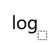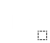ln
(
)
7
8
9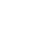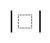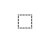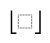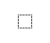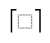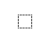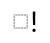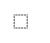τ
π
4
5
6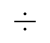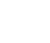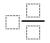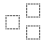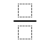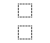%
θ
1
2
3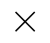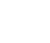<
>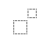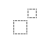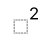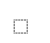x
i
0
.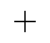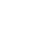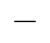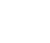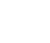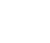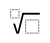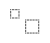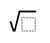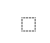y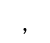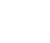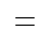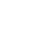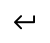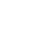Solve for rSteps for Solving Linear Equation
Multiply both sides of the equation by .
Subtract from both sides.
Combine and to get .
Add to both sides. Anything plus zero gives itself.
Divide both sides by .
Fraction can be rewritten as by extracting the negative sign.View solution steps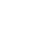Powered by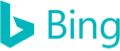r-3=8r
Multiply both sides of the equation by 4.
r-3-8r=0
Subtract 8r from both sides.
-7r-3=0
Combine r and -8r to get -7r.
-7r=3
Add 3 to both sides. Anything plus zero gives itself.
r=\frac{3}{-7}
Divide both sides by -7.
r=-\frac{3}{7}
Fraction \frac{3}{-7}\approx -0.428571429 can be rewritten as -\frac{3}{7}\approx -0.428571429 by extracting the negative sign.